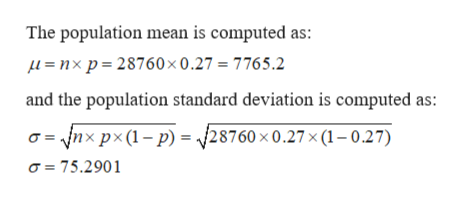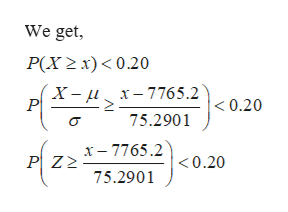# A crowd of 28,760 fans will be attending a soccer game. On average 27% of attendees will want to buy a meat pie. Use a normal distribution to find how many meat pies the stadium should order to ensure a less than 20% chance of running out of meat pies. (Note that no σ has been provided. To determine it, we assume that we are approximating a binomial distribution in which σ 2 = np(1−p). Moreover, to correct for issues in approximating a discrete quanity (people) using a continuous distribution, it is normal to subtract 0.5 from lower bounds and add 0.5 to upper bounds.)

Question
1 views

A crowd of 28,760 fans will be attending a soccer game. On average 27% of attendees will want to buy a meat pie. Use a normal distribution to find how many meat pies the stadium should order to ensure a less than 20% chance of running out of meat pies. (Note that no σ has been provided. To determine it, we assume that we are approximating a binomial distribution in which σ 2 = np(1−p). Moreover, to correct for issues in approximating a discrete quanity (people) using a continuous distribution, it is normal to subtract 0.5 from lower bounds and add 0.5 to upper bounds.)

check_circle

Step 1

Given,

population proportion of success is P = 27% = 0.27

n = Number of fans = 28760

Step 2

We have to find x where, P(X ≥ x) < 0.20

Here,help_outlineImage TranscriptioncloseThe population mean is computed as: L=nxp 28760 x 0.27 = 7765.2 and the population standard deviation is computed as: Vnx px (1p) /28760 x 0.27 x (1 -0.27) o= 75.2901 fullscreen
Step 3help_outlineImage TranscriptioncloseWe get P(X2x) 0.20 P x 7765.2 75.2901 X- < 0.20 x-7765.2 P Z2 <0.20 75.2901 fullscreen

### Want to see the full answer?

See Solution

#### Want to see this answer and more?

Solutions are written by subject experts who are available 24/7. Questions are typically answered within 1 hour.*

See Solution
*Response times may vary by subject and question.
Tagged in

### Other